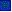## A 21st Century Lens on Artificial IntelligenceMathematician develops equations that seek to bridge the micro and macro realms (February 7, 2023))YT Talk: Weinan E: A Mathematical Perspective on Machine Learning (July 8, 2022)Stephen Wolfram: " Finally We May Have a Path to the Fundamental Theory of Physics and Its Beautiful (April 14, 2020)Lex Fridman interview with Donald Knuth: Algorithms, Complexity, Life, and The Art of Computer Programming | AI Podcast (December 30, 2019)Wiki: CalculusNature: Simple artificial-intelligence problem puts researchers up against a logical paradox discovered by famed mathematician Kurt G del. (January 8, 2019)MIT OpenCourseware: Lecture: Mathematics of Big Data and Machine Learning (November 9, 2018)Institute for Quantum Computing: A Universal Training Algorithm for Quantum Deep Learning (October 15, 2018)The Mathematics of Machine Learning (July 8, 2016)Differentiable Programming (December 2015)Why Machine Learning needs statistics (December 2015)Causal Thinking in the Twilight Zone Judea Pearl (July 2015)Claimed Breakthrough Slays Classic Computing Problem; Encryption Could Be Next (November 2015)Critical Algorithm Studies: a Reading List (November 2015)New general-purpose optimization algorithm promises order-of-magnitude speedups on some problems (October 2015)P vs. NP and the Computational Complexity ZooAvi Wigderson on the "P vs. NP" problem - Efficient computation, internet security & the limits to human knowledgeMajor advance reveals limits of computation - Maybe?Sebastian Seung's Quest to Map the Human Brain - MathematicsAlgorithms for the Satisfiability (SAT) Problem: A Survey (1996)Amoeba-inspired computing system outperforms conventional optimization methodsComplexity No Bar to AIStephen Wolfram: Computation and the Future of MathematicsStephan Wolfram: Is Mathematics Invented or DiscoveredStephan Wolfram:The Background and Vision of MathematicaAaron Sloman: "Evolution, robots and mathematics"Common Probability Distributions: The Data Scientist s Crib Sheet (December 2015)Gunnar Carlsson: The Shape of Data - Topological Mapping (September 2015)The Banff International Research Station (BIRS)Institute for Pure & Applied Mathematics (IPAM)Pacific Institute for the Mathematical Sciences (PIMS, Canada)Mathematical Sciences Research Institute (MSRI, Berkeley, USA)Institute of Applied Mathematics (UBC, Canada)Simons Foundation - Advancing Research in Basic Science and MathematicsWolfram MathWorldAustin Fowler: Quantum computing and the need for new algorithmsThe Achampong Theorem and national securityUnconventional Computing - beyond Von Neuman and Turing - MULTI-level logic variablesMethod to shortcut simulations to reach previously unreachable timescales & ID practicable problemsGodel's Lost Letter and P=NP (with Blogroll)Efficient discovery of overlapping communities in massive networksThe Baffling and Beautiful Wormhole Between Branches of Math - Euler s identityLive Fourier Transform demo, showing small-angle scattering patterns for some structuresLook at Nothing - Blog on small-angle scatteringStanford University: Lecture 1 | The Fourier Transforms and its ApplicationsScott Aaronson - Thought experiments: Is There Anything Beyond Quantum Computing?Primes - a visual tribute The Riemann HypothesisPerhaps we can rigorously develop a science of when large sections of proofs can be effectively handwavedThe real 10 algorithms that dominate our worldWolfram MathworldAlgorithmia - Open Marketplace for AlgorithmsAxiomatic method is not fully adequate to the current mathematical practice arguedInternational Mathematical UnionClay Mathematics InstituteSimons FoundationDusa McDuffThe research interests of Henry CohnGil Press - A short history of digitizationEncyclopedia of Integrer Sequences (OEIX) - SloaneSee Also the Artificial Intelligence Section of CascadiaprimeSee Also the Quantum Computing Section of CascadiaprimeSee Also the Neuroscience Section of Cascadiaprime